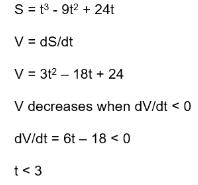# Test: Application Of Derivative (Competition Level) - 2

## 30 Questions MCQ Test Mathematics (Maths) Class 12 | Test: Application Of Derivative (Competition Level) - 2

Description
This mock test of Test: Application Of Derivative (Competition Level) - 2 for JEE helps you for every JEE entrance exam. This contains 30 Multiple Choice Questions for JEE Test: Application Of Derivative (Competition Level) - 2 (mcq) to study with solutions a complete question bank. The solved questions answers in this Test: Application Of Derivative (Competition Level) - 2 quiz give you a good mix of easy questions and tough questions. JEE students definitely take this Test: Application Of Derivative (Competition Level) - 2 exercise for a better result in the exam. You can find other Test: Application Of Derivative (Competition Level) - 2 extra questions, long questions & short questions for JEE on EduRev as well by searching above.
QUESTION: 1

### The value of ‘a’ for which x3 - 3x + a = 0 has two distinct roots in [0, 1] is given by

Solution:

Let α, β ∈[0,1].f (x) is continuous on [a,b] & differentiable on (a,b) and f (α) = f (β) = 0
∴ c ∈ (α, β) such that f' (c) = 0 ⇒ c = ±1∉ (0,1)

QUESTION: 2

### The value of ‘c’ in Lagrange’s mean value theorem for f (x) = x (x- 2)2 in [0, 1]

Solution:

f '(c) = 0 2c(c - 2) + (c - 2)2 = 0
c = 2,2/3
∴ c = 2/3 (c ≠2)

QUESTION: 3

### For the function f (x) = x3 - 6x2 + ax + b, if Roll’s theorem holds in [1, 3] with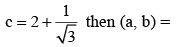Solution:

f (1) = f (3) ⇒ a = 11

QUESTION: 4

Find Value of ‘c’ by using  Rolle’s theorem for f (x) = log (x2 + 2) - log 3 on [-1,1]

Solution: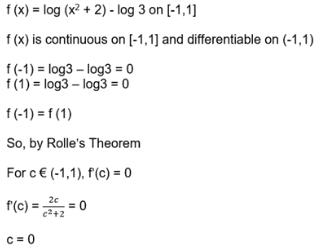QUESTION: 5

The chord joining the points where x = p and x = q on the curve y = ax2 + bx + c is parallel to the tangent at the point on the curve whose abscissa is

Solution:

Apply Lagrange’s theorm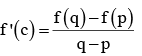QUESTION: 6

The least value of k for which the function f(x) = x2 + kx + 1 is a increasing  function in the interval 1 < x < 2

Solution: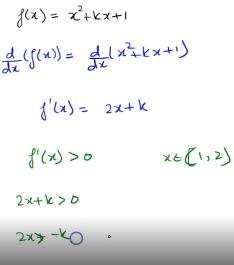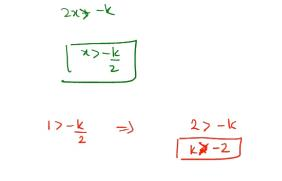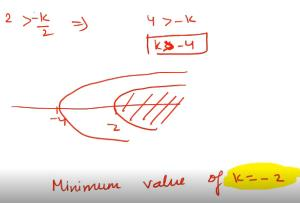QUESTION: 7

The interval in  which f (x) = x3 - 3x2 - 9x + 20 is strictly decreasing

Solution:

Given f (x) = x3 - 3x2 - 9x + 20
⇒ f '(x) = 3x2 -6x -9
⇒ f '(x) = 3(x - 3)(x +1)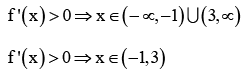Thus, f (x) is strictly increasing for
x ∈ (-∞,-1) U (3, ∞) and strictly decreasing for x ∈ (-1, 3)

QUESTION: 8

The critical points of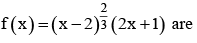Solution: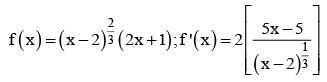f ' (x) = 0 ⇒ x = 1; f1 (x) does not exist at x = 2
∴ x = 1 and x = 2 are two critical points

QUESTION: 9

The number of stationary points of f (x) = sin x in [0,2π] are

Solution:

f (x) = sinx ⇒ f '(x) = cosx ⇒ f '(x) = 0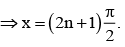Therefore number of stationary points of f (x) in [0, 2π] is 2.

QUESTION: 10

Local minimum values of the function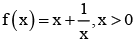Solution:

AM > GM

QUESTION: 11

If the function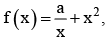has maximum at x =-3, then the value of ‘a’ is

Solution: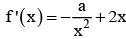since f (x) has local maximum at x = -3 ⇒ f ' (-3) = 0 and f 11 (-3)< 0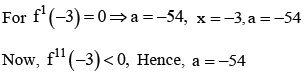QUESTION: 12

The point at which f (x) = (x- 1)4 assumes local maximum or local minimum value are

Solution: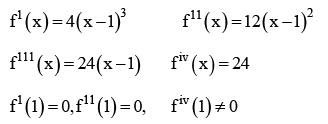Therefore n = iv is even and fiv (1) = 24> 0
Therefore f (x) has local minimum at x = 1.

QUESTION: 13

The global maximum and global minimum of f (x) = 2x3 - 9x2 + 12x + 6 in [0, 2]

Solution: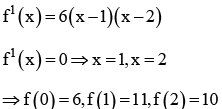Therefore global maximum M1 = max{f (0), f (1), f (2)}= 11
Global minimum
M2 = max{f (0), f (1), f (2)}= 6

QUESTION: 14

The approximate value of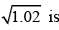Solution: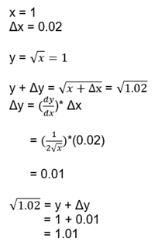QUESTION: 15

The approximate value of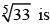Solution: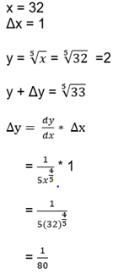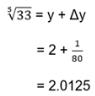QUESTION: 16

If the percentage error in the surface area of sphere is k, then the percentage error in its volume is

Solution: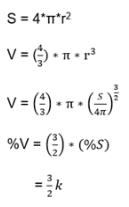QUESTION: 17

If an error of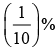is made in measuring the radius of a sphere then percentage error in its volume is

Solution:

V% = 3(S%)

QUESTION: 18

The height of a cylinder is equal to its radius. If an error of 1 % is made in its height. Then the percentage error in its volume is

Solution:

h = r and v = ph3; V% = 3( h%)

QUESTION: 19

The slope of the normal to the curve given by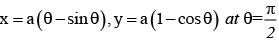Solution: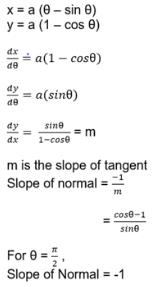QUESTION: 20

The line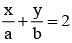is a tangent to the curve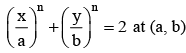then n ∈

Solution:

Calculate slope

QUESTION: 21

The points on the curve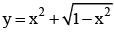at which the tangent is perpendicular to x-axis are

Solution:

dy/dx is not defined.

QUESTION: 22

The point on the curve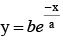at which the tangent drawn is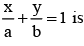Solution:
QUESTION: 23

The sum of the squares of the intercepts on the axes of the tangent at any point on the curve x 2/3 + y2/3= a2/3 is

Solution:

Equation of the tangent at p (θ) to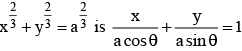QUESTION: 24

If the straight line x cos α + y sinα = p touches the curve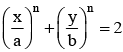at the point (a, b) on it, then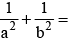Solution:

Find dy/dx and the equation of the tangent

QUESTION: 25

If the curves x = y² and xy = k cut each other orthogonally then k² =

Solution:

m1.m2 =-1

QUESTION: 26

The angle between the curves y = x³ and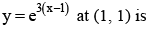Solution:

Find dy/dx to the two curves at (1, 1) they are m1 and m2. Then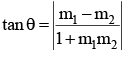QUESTION: 27

If the curves ay + x² = 7 and x³ = y cut orthogonally at (1, 1) then a =

Solution:

Slope of the first curve at (1, 1) is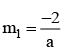Slope of the second curve at (1, 1) is m2 = 3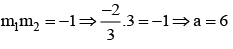QUESTION: 28

A particle moves along a line is given by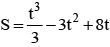then the distance travelled by the particle before it first comes to rest is

Solution: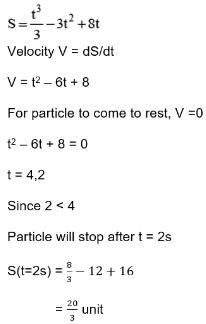QUESTION: 29

A particle is moving along a line such that s = 3t3 - 8t + 1. Find the time ‘t’ when the distance ‘S’ travelled by the particle increases.

Solution: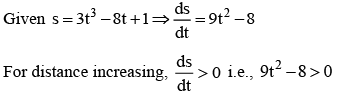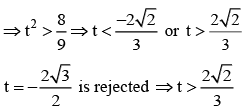QUESTION: 30

A particle moves along a line by S = t3 - 9t2 + 24t the time when its velocity decreases.

Solution: